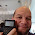## Thursday, May 3, 2012

### Calculus Revisited #11: Integration by Substitution

Welcome to Part 11 of our 21-part Calculus Revisited journey! Today we are continuing with integrals - and starting the first of four blog entries concerning techniques of integration. I will try my best to explain each one but I feel the best way to demonstrate these techniques is by example.

Integration by Substitution

We have the integral ∫ f(x) dx.

Find a function u(x) such that

du = u'(x) dx and

∫ f(u) * du is easier to integrate than ∫ f(x) dx.

Then ∫ f(u) du = F(u) + C

For the definite integral

b
∫ f(x) dx
a

du = u'(x) dx

and the integral is re-written as

u(b)
∫ f(u) du = F(u(b)) - F(u(a))
u(a)

Let's get to the solved problems to demonstrate this technique.

Problems
Indefinite Integrals

1. ∫ √(4 + 5x) dx

Let u(x) = 4 + 5x
Then du = 5 dx

Note that √u = √(4 + 5x)
and dx = du/5

Rewriting the integral:

∫ √(4 + 5x) dx
= ∫ √u * 1/5 du
= 1/5 * ∫ √u du
= 1/5 * (u^(3/2))/(3/2) + C
= 1/5 * 2/3 * u^(3/2) + C
= 2/15 * u^(3/2) + C
When substitution is used, please don't not forget to state the answer in the original variable, in this case, x. u = 4 + 5x
= 2/15 * (4 + 5x)^(3/2) + C

2. ∫ sin x / √(1 + 2 cos x) dx

Let u = 1 + 2 cos x
Then du = - 2 sin x dx

Note - du/2 = sin x dx (which matches the numerator)

Rewriting the integral:
∫ sin x / √(1 + 2 cos x) dx
= ∫ 1/√u * -1/2 du
= -1/2 * ∫ u^(-1/2) du
= -1/2 * u^(1/2) * 2 + C
= -u^(1/2) + C
= -√u + C
Remember... the answer must contain the original variable!
= -√(1 + 2 cos x) + C

The right substitutions make integration much easier. The next two problems illustrate this.

3. ∫ sin^4 x * cos x dx

Let u = sin x
Then du = cos dx (note the cos x in the integral)

∫ sin^4 x * cos x dx
= ∫ u^4 du
= u^5/5 + C
= sin^5 x/5 + C

4. ∫ e^x / (e^(2x) + 1) dx

Let u = e^x
Then du = e^x dx (matches the numerator)

∫ e^x / (e^(2x) + 1) dx
= ∫ du/(u^2 + 1)
= atan u + C
= atan (e^(2x)) + C

Definite Integrals

If you use the substitution technique with indefinite integrals, do NOT forget to account for the limits! For definite integrals, you will recalculate the limits to account for the substitution. The next two problems illustrate this point.

5. Calculate

1
∫ cos (2x) dx
-1

Let u = 2x
Then du = 2 dx

Note du/2 = dx (try to get the term with the dx to match some part of the integral)

Accounting for the limits:
New Upper Limit = 2 * 1 = 2
New Lower Limit = 2 * -1 = -2

Putting it together:

1
∫ cos (2x) dx
-1

2
= ∫ cos u * 1/2 du
-2

= 1/2 * (sin(2) - sin(-2))

Recall sin(-x) = -sin x

= 1/2 * (sin(2) + sin(2)) = sin(2) ≈ 0.90930

Finding the right substitution may be tricky at times. Algebraic manipulation may be necessary. Here is a more difficult problem.

6. Calculate

9
∫ √t / (√t - 1) dt
4

Let u = √t - 1
Then du = 1/(2√t) dt

To help match the integral, (2√t) du = dt

Note that 2√t = 2(u + 1) = 2u + 2 (Look at the substitution and solve for √t).

So (2u + 2) du = dt

We can't forget about the limits, since we are working with a definite integral.
u = √t - 1

New Upper Limit: √9 - 1 = 4 - 1 = 3
New Lower Limit: √4 - 1 = 2 - 1 = 2

Putting this together:

9
∫ √t / (√t - 1) dt
4

2
= ∫ (u + 1)/u * (2u + 2) du
1

2
= ∫ (1 + 1/u) * (2u + 2) du
1

2
= ∫ 2u + 2 + 2 + 2/u du
1

2
= ∫ 2u + 4 + 2/u du
1

The antiderivative is u^2 + 4u + 2 ln u

= ( (2)^2 + 4(2) + 2 ln (2) ) - ( (1)^2 + 4(1) + 2 ln (1) )

= ( 4 + 8 + 2 ln 2 ) - ( 1 + 4 + 2 * 0 )

= 7 + 2 ln 2 ≈ 8.38629

Best thing to do if you are in a calculus course is to practice, practice, practice!

I hope these six example problems give you an understanding of the substitution technique. Mastery of this technique will come with time and practice - and will speed up integral evaluation.

Next time, we look at the famous (or maybe infamous) Integration by Parts technique.

See you soon,

Eddie

This blog is property of Edward Shore. © 2012

1.Thanks for sharing this about Integral as-Common anti derivative that are used in rational functions are anti derivative of constant is âˆ« k dx = kx + c b) and anti derivative of power function is âˆ« xn dx = xn + n + 1 c)
Real Numbers Definition

2.You are most welcome harun.

3.Thanks. It's a nice post about integration by substitution. I really like it :). It's really helpful. Good job.

### Retro Review and Comparison: TI-82 Advanced

Retro Review and Comparison: TI-82 Advanced I can officially say that I have a French calculator.   Quick Facts: Models:  TI-82 Advanced Co...